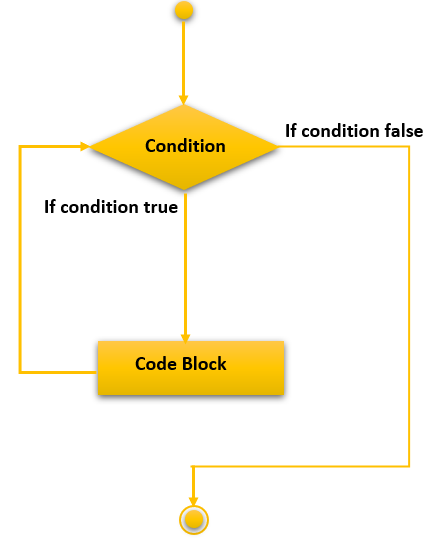# C++ While Loop

In this tutorial, you will learn about the while loop in the C++ programming language with the help of examples.

In C++, while loops are used when you do not know how many times a particular loop will execute. For loops are used when you know previously how many times a loop will execute. While loops are common where the program runs in an infinite loop until the user terminates it or while a specific input device will keep sending it input, the while statement will continue to execute.

### Syntax

The syntax for while loop is as follows:

```Initialization of the expression
while (test or exit condition expression)
{
// statements which will be executed when the condition is true
update_expression;
}```

On breaking down the different parts of a while loop we will get the following:

• Initialization Expression: This expression is used to initialize the while loop with an initial value by either declaring and initializing a variable there or only by initializing an existing variable at that point. It is declared outside the body of the loop. Eg. `int i=6`; or `j=k;` // Provided both `j` and `k` have been declared and `k` has been initialized.
• Test or Exit Condition: This expression will keep on getting executed until the condition is false and then the program flow will move out of the while loop. If the condition is true, it will move into the block and execute the statements inside the while loop. Eg. `i<10;`
• Update Expression: This expression will get executed after the statements in the while loop have been executed and before the while loop condition is evaluated. It will execute as many times as the while loop statement will get executed and it can be mentioned anywhere in the while block. Eg. `i++;`

## Flowchart of While Loop in C++## The Structure of While Loop Execution

The while loop execution follows the following pattern of code execution which are as follows :

1. Execution begins by initialization
2. The execution moves onto the condition of the while loop.
1. If the condition is true, the flow moves onto the body.
2. If the condition is false, the flow moves to the outside of the body of the loop.
3. The loop block and the update statement are executed.
4. After the update, the control moves to the condition statement in Step no 2.
5. The above statements from steps 4 to 2 are repeated till the condition is false. Once false it exits from the block.

### Example

```//A C++ program to show how a while loop works
#include <iostream>
using namespace std;
int main()
{
// Writing a for loop to print Hello World 4 times
int i=1;
while ( i<5 ){
cout<<"Hello World\n";
i++;
}
return 0;
}```

Hello World
Hello World
Hello World
Hello World

## Program Flow

1. The program starts its execution.
2. `i` is initialized with value 1.
3. Condition is checked. 1 <= 6 yields true.
a) “Hello World” gets printed 1st time.
b) Updation is done. Now `i = 2`.
4. Condition is checked. 2 <= 6 yields true.
a) “Hello World” gets printed 2nd time.
b) Updation is done. Now `i = 3`.
5. Condition is checked. 3 <= 6 yields true.
a) “Hello World” gets printed 3rd-time.
b) Updation is done. Now `i = 4`.
6. Condition is checked. 4 <= 6 yields true.
a) “Hello World” gets printed 4th-time
b) Updation is done. Now `i = 5`.
7. Condition is checked. 5 < 5 yields false.
8. The flow goes outside the loop to return 0.

## Nested While Loop

You can nest one while loop inside another by simply writing the second while loop code inside the first while loop block. For example, the following code will explain how a nested loop works.

```//A C++ program to show how a nested while loop works
#include <iostream>
using namespace std;
int main()
{
int j,i=1;
while ( i<=5 ){
while ( j<=5 ) {
j=i;
cout<<j;
j++;
}
cout<<endl;
i++;
}return 0;
}```

1 2 3 4 5
2 3 4 5
3 4 5
4 5
5

## Infinite While Loop

An infinite While loop can be due to a logical error or can be deliberately placed for a particular reason like waiting for the program to receive an input from a source and then continue execution. It can be achieved by either removing the exit condition or by not providing an update statement or even both.

### Example 1: Infinite while loop

```//A C++ program to show how an infinite loop works
#include <iostream>
using namespace std;
int main()
{
int i=1;
while ( i<5 ) { // No update statement
cout << "Hello World\n";
}
return 0;
}```

Hello World
Hello World
Hello World
Ctrl + C

### Example 2: Infinite while loop

```//A C++ program to show how an infinite loop works
#include <iostream>
using namespace std;
int main()
{
int i=1;
while ( i>0 ) { // Condition is always true
cout << "Hello World\n";
}
return 0;
}```

Hello World
Hello World
Ctrl + C